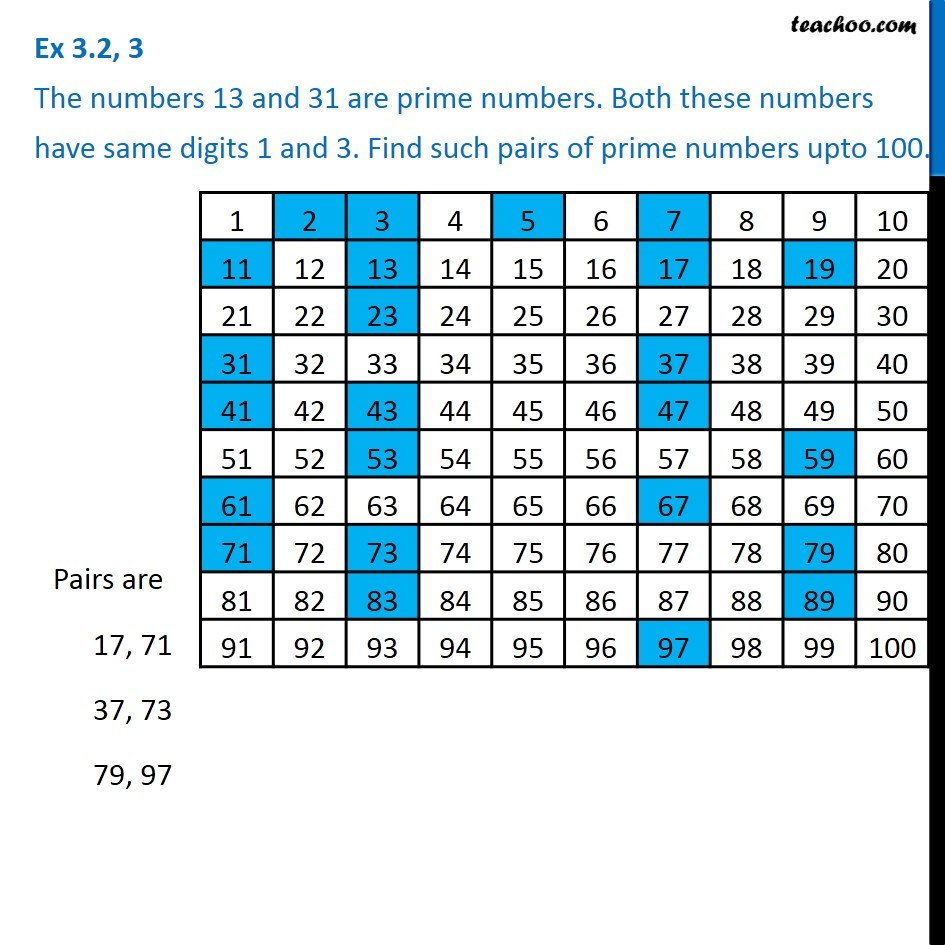Ex 3.2

Chapter 3 Class 6 Playing with Numbers
Serial order wise### Transcript

Ex 3.2, 3 The numbers 13 and 31 are prime numbers. Both these numbers have same digits 1 and 3. Find such pairs of prime numbers upto 100. Prime numbers are numbers which have only 2 factors - 1 and the number itself. Now, lets see a list of numbers from 1 to 100, and mark which are prime numbers. By seeing the list, the numbers which have the same digits, and are prime are 17, 71 37, 73 79, 97